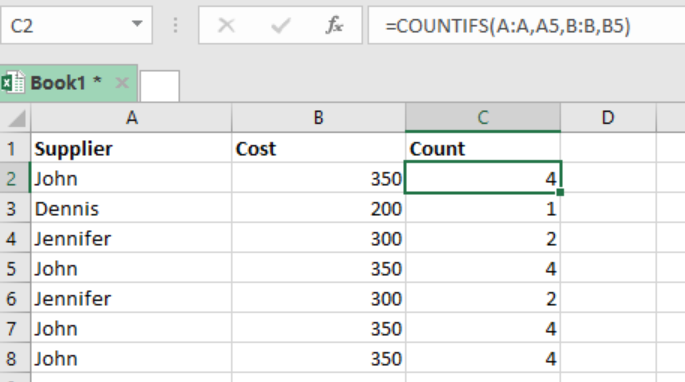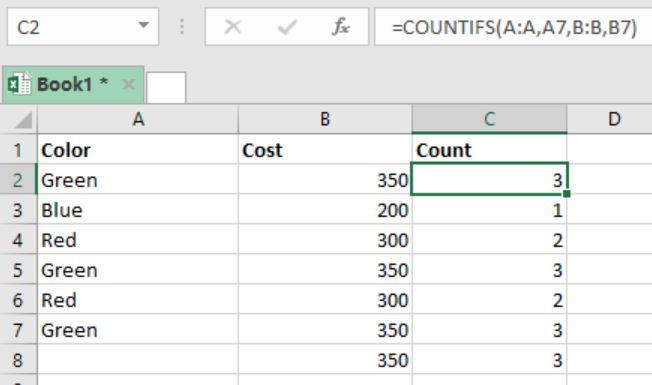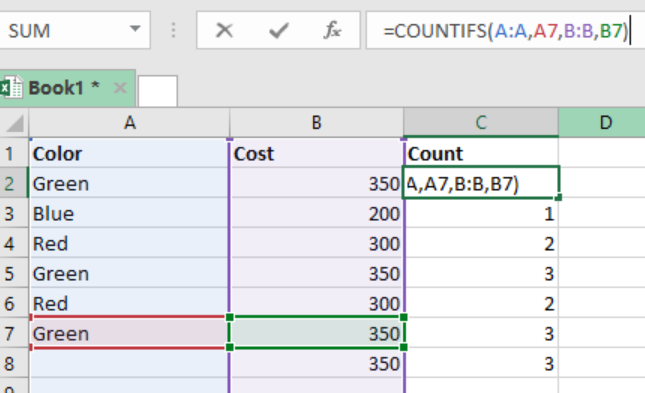Get instant live expert help with Excel or Google Sheets“My Excelchat expert helped me in less than 20 minutes, saving me what would have been 5 hours of work!”

#### Post your problem and you’ll get Expert help in seconds.

Your message must be at least 40 characters
Our professional Expert are available now. Your privacy is guaranteed.

# Count Items in a List

If we want to know the count of values that appear in a list or table, we can utilize the COUNTIFS function. This article will guide you on how to use the COUNTIFS function to quickly count items in a list.Figure 1. Final result

## Syntax of the formula

`=COUNTIFS (A:A, A1, B:B, B1)`

## How the formula works

•         COUNTIFS function works with a pair of range/criteria
•         If the all the given criteria match, then the function will give the count of all values in the given range.
•         The range is entered as a full column reference.

In the example above, we have two column reference, B:B and C:C. the criteria is as shown in cell C2, i.e. `=COUNTIFS(A:A,A5,B:B,B5)`

These two pair of column reference will appear in the COUNTIFS function, and will thus link the values of column B and C. The COUNTIFS will then generate the count of the values in both column references based on the supplied criteria.

## ExampleFigure 2. Setting up data

In the example above, we want to count the number of items in the excel sheet. Here is a step by step guide on how we got the counts in column C:

Step 1: prepare the data you want to work on

Step 2: Mark column C as “Count”, as that is where we shall have our counts

Step 3: In cell C2, specify the formula, `=COUNTIFS (A:A,A7,B:B,B7)`Figure 3. Input formula

Step 4: Press Enter to get the count of the first row.

Step 5: Copy down the formula to get the counts for the other rows

## Instant Connection to an Expert through our Excelchat Service

Most of the time, the problem you will need to solve will be more complex than a simple application of a formula or function. If you want to save hours of research and frustration, try our live Excelchat service! Our Excel Experts are available 24/7 to answer any Excel question you may have. We guarantee a connection within 30 seconds and a customized solution within 20 minutes.

### Did this post not answer your question? Get a solution from connecting with the expert.Another blog reader asked this question today on Excelchat:
Solution examplesi need a formula to find values in column f in worksheet 1 and count them.
Solved by B. A. in 20 minsI have a spreadsheet that contains a list of names/IDs and I want to generate a formula that inserts into a cell the number of times a name or ID appears on that list. Either insert a column into that spreadsheet or transfer that info into a column on a different spreadsheet. Either way would be fine.
Solved by O. L. in 18 minsi need a formula to count values that meet two conditions: one if a value is contained in a cell and another one is cells aren't empty
Solved by X. Q. in 20 minsGreetings, I am trying to find a formula in excel that will return the number of students attending courses. My data is on one sheet and my report will be on another sheet. The report is formated with the students list in a coulmn (far left), the courses are list in a row (top). My data is formatted with all columns, courses in column in "A" and students in column "B". Thanks
Solved by E. C. in 27 minsin range b2:o2, I am trying to write a formula to count if the values are equal to 1 thru 27.
Solved by E. L. in 52 mins## Subscribe to Excelchat.coAnother blog reader asked this question today on Excelchat: